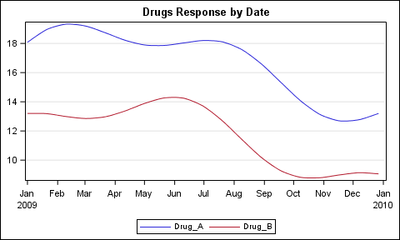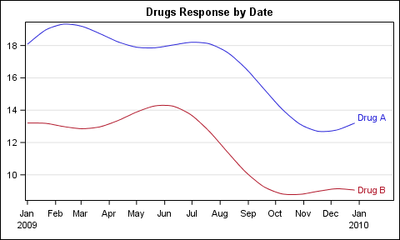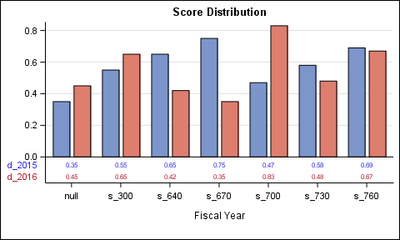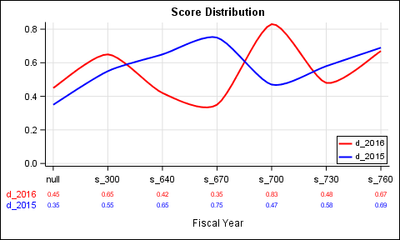## how to draw two smooth curves in sas

Hello,

I have the code below which draws two curves, but I want them to be smooth lines, without any dots. Would you please help me? Thanks!

libname reflib 'SAS-data-library';
goptions reset=global gunit=pct border cback=white
colors=(black blue green red)
ftitle=swissb ftext=swiss htitle=6 htext=4;

title1 'Dow Jones Yearly Highs and Lows';
footnote1 h=3 j=l ' Source: 1997 World Almanac'
j=r 'GR21N06 ';

symbol1 color=red
interpol=join
value=dot
height=3;
symbol2 font=marker value=C
color=blue
interpol=join
height=2;

axis1 order=(1955 to 1995 by 5) offset=(2,2)
label=none
major=(height=2) minor=(height=1)
width=3;

axis2 order=(0 to 6000 by 1000) offset=(0,0)
label=none
major=(height=2) minor=(height=1)
width=3;

legend1 label=none
shape=symbol(4,2)
position=(top center inside)
mode=share;

proc gplot data=reflib.stocks;
plot high*year low*year / overlay legend=legend1
vref=1000 to 5000 by 1000 lvref=2
haxis=axis1 hminor=4
vaxis=axis2 vminor=1;
run;
quit;

10 REPLIES 10

## Re: how to draw two smooth curves in sas

Use PROC SGPLOT (base SAS) instead of PROC GPLOT (SAS/GRAPH).

See these 2 blogs from Rick Wicklin.

Three ways to add a smoothing spline to a scatter plot in SAS

Rick Wicklin | FEBRUARY 24, 2014

How to automatically select a smooth curve for a scatter plot in SAS
Rick Wicklin | FEBRUARY 26, 2014

http://blogs.sas.com/content/iml/2014/02/26/how-to-automatically-select-a-smooth-curve-for-a-scatter...

Cheers,

Koen

## Re: how to draw two smooth curves in sas

Hi,

To update the code as below, which I got two lines but with dots and triangles, do you know how to remove the dots and triangles and make the two curves smooth? thanks!

goptions reset=all border;
data stocks;
input year high low @@;

datalines;
1956 521.05 462.35 1957 520.77 419.79
1958 583.65 436.89 1959 679.36 574.46
1960 685.47 568.05 1961 734.91 610.25
1962 726.01 535.76 1963 767.21 646.79
1964 891.71 768.08 1965 969.26 840.59
1966 995.15 744.32 1967 943.08 786.41
1968 985.21 825.13 1969 968.85 769.93
1970 842.00 631.16 1971 950.82 797.97
1972 1036.27 889.15 1973 1051.70 788.31
1974 891.66 577.60 1975 881.81 632.04
1976 1014.79 858.71 1977 999.75 800.85
1978 907.74 742.12 1979 897.61 796.67
1980 1000.17 759.13 1981 1024.05 824.01
1982 1070.55 776.92 1983 1287.20 1027.04
1984 1286.64 1086.57 1985 1553.10 1184.96
1986 1955.57 1502.29 1987 2722.42 1738.74
1988 2183.50 1879.14 1989 2791.41 2144.64
1990 2999.75 2365.10 1991 3168.83 2470.30
1992 3413.21 3136.58 1993 3794.33 3241.95
1994 3978.36 3593.35 1995 5216.47 3832.08
;
title1 "Dow Jones Yearly Highs and Lows";
footnote1 j=l " Source: 1997 World Almanac"
;
symbol1 interpol=join
value=dot
color=_style_;
symbol2 interpol=join
value=C
font=marker
color=_style_ ;
axis1 order=(1955 to 1995 by 5) offset=(2,2)
label=none
major=(height=2)
minor=(height=1)
;

axis2 order=(0 to 6000 by 1000) offset=(0,0)
label=none
major=(height=2)
minor=(height=1)
;
legend1 label=none
position=(top center inside)
mode=share;
proc gplot data=stocks;
plot high*year low*year / overlay legend=legend1
vref=1000 to 5000 by 1000
lvref=2
haxis=axis1 hminor=4
vaxis=axis2 vminor=1;
run;
quit;

## Re: how to draw two smooth curves in sas

That's easy.

In your 2 symbol statements, use value=NONE (no markers).

In your 2 symbol statements, use a spline interpolation method instead of interpol=join (which asks for a straight line segment to connect the adjacent measurements).

spline interpolation methods

 INTERPOL=L

INTERPOL=SM

INTERPOL=SPLINE

Cheers,

Koen

## Re: how to draw two smooth curves in sas

To be even more specific, here are the two SYMBOL statements you need:

symbol1 interpol=sm
value=none
color=_style_;

symbol2 interpol=sm
value=none
font=marker
color=_style_ ;

For more smoothing, put sthg. like:

symbol1 interpol=sm45
value=none
color=_style_;

symbol2 interpol=sm45
value=none
font=marker
color=_style_ ;

SYMBOL INTERPOL=SMnn option:

Good luck,

KoenJay54
Meteorite | Level 14

## Re: how to draw two smooth curves in sas

With SGPLOT, this is quite easy.  See attached code below.  Note the data is at 20 unit intervals.  SMOOTHCONNECT can create a smooth join.  You can have legend or curve labels.  You can add MIN=0 to YAXIS of you want to start y-axis from zero.

```/*--MultiSeries Data--*/
data MultiSeries;
keep Date Drug_A Drug_B;
format Date Date9.;
do i=0 to 364 by 20;
date='01jan2009'd+i;
Drug_A= 16+ 3*sin(i/90+0.5) + 1*sin(3*i/90+0.7);
Drug_B= 11+ 3*sin(i/90+0.5) + 1*cos(3*i/90+0.7);
output;
end;
run;

/*--With Legend--*/
ods listing;
ods graphics / reset attrpriority=color width=5in height=3in imagename='MultiSeries';
title 'Drugs Response by Date';
proc sgplot data=multiseries;
series x=date y=drug_a / smoothconnect;
series x=date y=drug_b / smoothconnect;
xaxis display=(nolabel);
yaxis display=(nolabel) grid;
run;

/*--With Curve Labels--*/
ods graphics / reset attrpriority=color  width=5in height=3in imagename='MultiSeries_Label';
title 'Drugs Response by Date';
proc sgplot data=multiseries;
series x=date y=drug_a / smoothconnect curvelabel='Drug A';
series x=date y=drug_b / smoothconnect curvelabel='Drug B';
xaxis display=(nolabel);
yaxis display=(nolabel) grid;
run;```## Re: how to draw two smooth curves in sas

Hi Sanjay,

Would you please suggest me how to change the code below, so that I could get a two smooth curves instead of the bars. I need to keep the boxes showing percentages below as the graph shows. Thank you so much!

goptions reset=all cback=white border htitle=12pt htext=10pt;

data ds1;
input year \$ mid \$ resp exp rev;
datalines;
null d_2016 0.45 0.45 0.35
null d_2015 0.35 0.45 0.35
s_300 d_2016 0.65 0.65 0.55
s_300 d_2015 0.55 0.65 0.55
s_640 d_2016 0.42 0.42 0.65
s_640 d_2015 0.65 0.42 0.65
s_670 d_2016 0.35 0.35 0.75
s_670 d_2015 0.75 0.35 0.75
s_700 d_2016 0.83 0.83 0.47
s_700 d_2015 0.47 0.83 0.47
s_730 d_2016 0.48 0.48 0.58
s_730 d_2015 0.58 0.48 0.58
s_760 d_2016 0.67 0.67 0.69
s_760 d_2015 0.69 0.67 0.69
;
run;

data ds2;
set ds1;
n=_n_;
run;

data _null_;
set ds2 end=eof;
if eof then call symput('skip',left(n));
run;

proc sort;
by year mid;
run;

data anno;
set ds2;
by year mid;
length function color \$8 text \$20 style \$ 20;

/* Populate the table */
if first.year then do;
function='move'; xsys='2'; ysys='1';
midpoint=mid; group=year; y=0;
output;
function='cntl2txt'; output;
function='label'; xsys='A'; ysys='3';
x=+2; y=13;
text=trim(left(put(exp,8.1)));
color='black'; position='+'; when='a';
output;
function='move'; xsys='2'; ysys='1';
midpoint=mid; group=year; y=0;
output;
function='cntl2txt'; output;
function='label'; xsys='A'; ysys='3';
x=+2; y=9;
text=trim(left(put(rev,8.1)));
color='black'; position='+'; when='a';
output;
end;

/* Generate the table frame */
function='move'; xsys='3'; ysys='3';
x=3; y=6;
output;
function='bar'; xsys='1'; ysys='3';
x=100; y=15;
style='empty'; color='black'; line=0;
output;

/* Generate the row headers */
function='label'; xsys='3'; ysys='3';
style='marker'; text='U'; color='cx7c95ca';
x=3; y=13; position='6';
output;
function='label'; xsys='3'; ysys='3';
style='"Albany AMT"'; text='d_2015'; color='black';
x=6; y=13; position='6';
output;
function='label'; xsys='3'; ysys='3';
style='marker'; text='U'; color='cxde7e6f';
x=3; y=8.5; position='6';
output;
function='label'; xsys='3'; ysys='3';
style='"Albany AMT"'; text='d_2016'; color='black';
x=6; y=9; position='6';
output;

/* Generate the vertical lines in the table */
function='move'; xsys='1'; ysys='1';
x=0; y=0;
output;
function='draw'; xsys='1'; ysys='3';
x=0; y=6;
line=1; color='black';
output;
function='move'; xsys='1'; ysys='1';
x=100; y=0;
output;
function='draw'; xsys='1'; ysys='3';
x=100; y=6;
line=1; color='black';
output;

if first.year and n ^=&skip then do;
function='move'; xsys='2'; ysys='1';
midpoint=mid; y=0; group=year;
output;
function='move'; xsys='A'; ysys='1';
x=+15.5; x=+8; y=0;
output;
function='draw'; xsys='A'; ysys='3';
x=+0; y=6;
color='black'; line=1;
output;
end;

/* Generate the horizontal line in the table */
function='move'; xsys='3'; ysys='3';
x=3; y=10.5;
output;
function='draw'; xsys='1'; ysys='3';
x=100; y=10.5;
line=1; color='black';
output;
run;

title1 'Score distribution';

axis1 label=(a=90 'Percentegy')
order=(0 to 1 by 0.1)
minor=none;

axis2 label=none
value=none
origin=(20pct,22pct)
offset=(4pct,4pct);

axis3 label=none;

footnote1 'Fiscal Year';
footnote2 h=.5 ' ';

pattern1 value=solid color=cx7c95ca;
pattern2 value=solid color=cxde7e6f;

proc gchart data=ds2;
vbar mid / sumvar=resp group=year
coutline=black patternid=midpoint
space=0 gspace=0 width=5
cframe=ltgray autoref clipref
raxis=axis1 maxis=axis2 gaxis=axis3
annotate=anno;
run;
quit;Jay54
Meteorite | Level 14

## Re: how to draw two smooth curves in sas

If you have SAS 9.4, this is very easy, and does not require any annotation at all.  Bar chart and Series Plot versions are shown below.  Note, a STYLEATTRS is added to the Series plot code to reverse the blue and red colors to match the bar chart.  Axis Table can be inside the axes (Bar) or outside (Series).  VLINE cannot be used as you want smoothconnect.  If you have a release prior ot 9.4, you will need to use SCATTER with MARKERCHAR or SGAnnotate to draw the data table.

It is important to emphasize that even though the X variable is "Year", the values in this example are character, not numbers.  The x-axis is not linear, so using a series plot for the response gives a false indication of "slope" between x values.  A series plot may not be appropriate in such cases, and the bar chart may be better.

```data ds1;
input year \$ mid \$ resp exp rev;
datalines;
null d_2016 0.45 0.45 0.35
null d_2015 0.35 0.45 0.35
s_300 d_2016 0.65 0.65 0.55
s_300 d_2015 0.55 0.65 0.55
s_640 d_2016 0.42 0.42 0.65
s_640 d_2015 0.65 0.42 0.65
s_670 d_2016 0.35 0.35 0.75
s_670 d_2015 0.75 0.35 0.75
s_700 d_2016 0.83 0.83 0.47
s_700 d_2015 0.47 0.83 0.47
s_730 d_2016 0.48 0.48 0.58
s_730 d_2015 0.58 0.48 0.58
s_760 d_2016 0.67 0.67 0.69
s_760 d_2015 0.69 0.67 0.69
;
run;
/*--Bar Chart--*/
ods listing gpath='.';ods graphics / reset attrpriority=color width=5in height=3in imagename='BarGroup';title 'Score Distribution';proc sgplot data=ds1 noborder noautolegend; vbar year / response=resp group=mid groupdisplay=cluster              outlineattrs=(color=black) barwidth=0.8; xaxistable resp / colorgroup=mid location=inside;  xaxis display=(nolabel); yaxis display=(nolabel) grid;run;/*--Series Plot--*/ods graphics / reset attrpriority=color width=5in height=3in imagename='SeriesGroup';title 'Score Distribution';proc sgplot data=ds1 noborder; styleattrs datacontrastcolors=(red blue); series x=year y=resp / group=mid smoothconnect         lineattrs=(thickness=2);  xaxistable resp / class=mid colorgroup=mid;  xaxis display=(nolabel) grid; yaxis display=(nolabel) grid min=0; keylegend / title='' linelength=20 across=1        location=inside position=bottomright ;run;
```## Re: how to draw two smooth curves in sas

Hi Sanjay,

Thank you so much for your help. Is it possible that the two graphs below is combined into one, which mean, the two curves and the bars are in the same picture, and the  boxes showing percentage are added into four rows, but the band of null,s_300,s_640,s_670,s_700,S_730.....should be top of  the four boxes showing percentage instead of being in the bottom, for this part, the second graph is preferred to the first graph. Second thing, could the small frame box on the right side corner of the second graph showing color and markers be moved down to replace the d_2015, d_2016 that is ahead of the percentage data. Third thing, could the percentage data rows below the graph are framed. Thank you very much!Jay54
Meteorite | Level 14

## Re: how to draw two smooth curves in sas

Yes, the plot statements can be combined.  If you want to overlay the series on the bars, use VBARPARM instead of VBAR.

Regarding the other details you want, I believe the goal of this communities forum is to point users in the right direction so you can then learn the tools to solve the problems on your own.  Doing the detailed graph for you sounds more like a consulting project.You can start from the code I have provided, and mix and match various statements to get the result you want.  It will be useful to consul the online doc, or other resources available on the web such as http://blogs.sas.com/content/graphicallyspeaking/.DanH_sas
SAS Super FREQ

## Re: how to draw two smooth curves in sas

The VALUE=DOT on the SYMBOL statment is putting the dots on your plot. Just remove this option. Do consider creating this plot using  PROC SGPLOT, as suggested by the other posters. You'll probably find it a lot easier to see how the options are applied.

Hope this helps!

Dan

Discussion stats
• 10 replies
• 5129 views
• 3 likes
• 4 in conversation